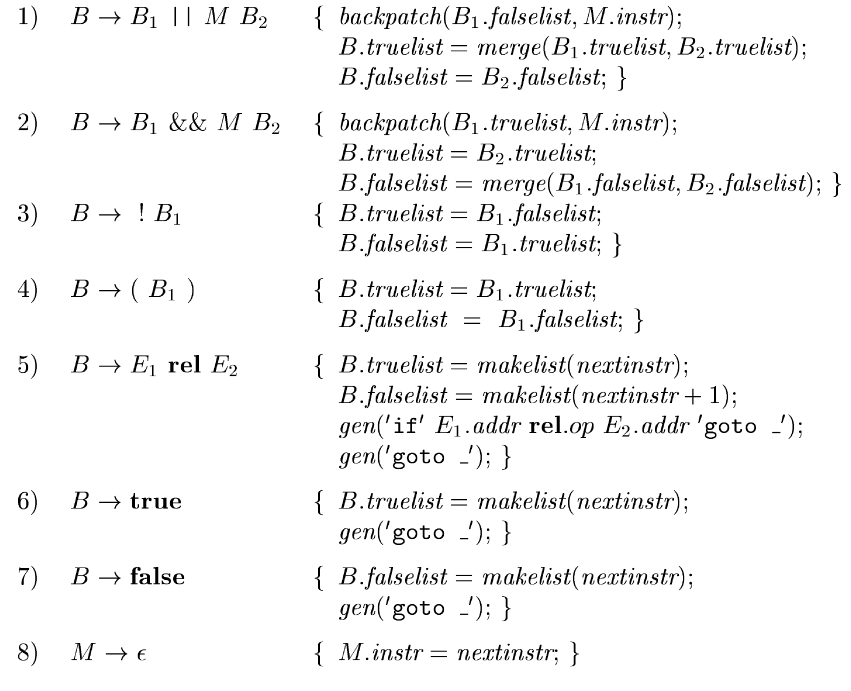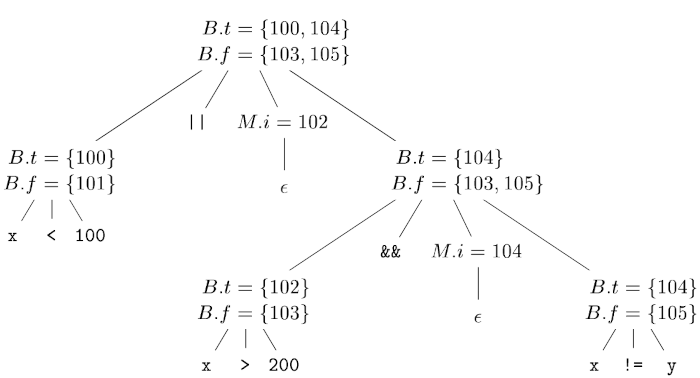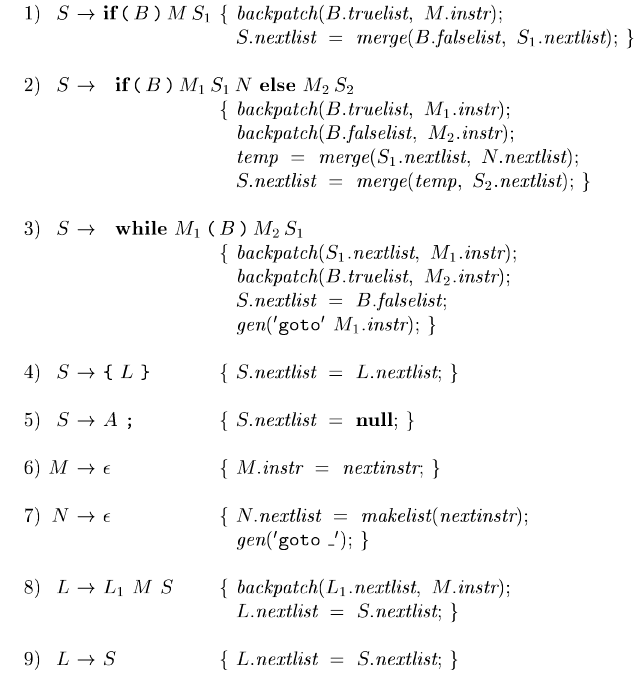×

Search anything:

# Backpatching

#### Compiler DesignFREE BOOK -> Problems for the day before your Coding Interview (on Amazon)

During the code generation phase, the compiler has to make jumps, however, the values needed for these jumps may not be known in a single pass therefore it improvises by filling up values which are replaced when the real values are known, a process known as backpatching.

1. Introduction.
2. One pass code generation.
3. Backpatching for boolean expressions.
4. Flow-control statements.
5. Break, continue and goto statements.
6. Summary.
7. References.

## Introduction.

An issue with generating code for boolean expressions or flow control statements involves matching a jump instruction with the target of the jump.

An example:
The translation of a boolean expression (B) S contains a jump when B is false , to instruction following the the code for S.
During a one-pass translation, B is translated before S is examined therefore this begs the question, what is the target of the goto that jumps over the code for S?

This problem can be solved by passing labels as inherited attributes where relevant jump instructions were previously generated, although for this we need a separate pass, In the following sections, we shall look at a complementary approach referred to as backpatching.

In backpatching, lists of jumps are passed as synthesized attributes.
When a jump is generated, the target of the jump is left unspecified. Such jumps are placed in a list of jumps whose labels are to be filled when a label can be determined.
All jumps in the list will have the same target label

## One-pass code generation.

Backpatching is used to generate code for boolean expressions and flow control statements.
With backpatching synthesized attributes truelist and falselist of a nonterminal B are used to manage labels in the jumping code for boolean expressions.

That is, B.truelist is a list of jump or conditional jump instructions into which we insert the label that control goes to if B is true and B.falselist is a list of instructions that gets the label to which control goes to when B is false.

During code generation for B, jumps to true and false exits stay incomplete with the label unfilled, these jumps are then placed in a list pointed to by B.truelist and B.falselist.

In a similar manner a statement S has a synthesized attribute s.nextlist that denotes a list of jumps to the instruction following the code for S.

To manipulate the lists we use the following three functions:

1. makelist(i) to create a new list with only an index i into the array of instructions. It returns a pointer to the new list.
2. merge(p1, p2), to concatenate the lists pointed to by p1 and p2 and return a pointer to this list.
3. backpatch(p, i) to insert i as the target label for each instruction on the list pointer to by p.

## Backpatching Boolean expressions.

We construct a translation scheme for generating code for boolean expressions during bottom-up parsing.
We have the following grammar:

B → ${\mathrm{B}}_{1}$ || M${\mathrm{B}}_{2}$ | ${\mathrm{B}}_{1}$ && M${\mathrm{B}}_{2}$ | !${\mathrm{B}}_{1}$ | !(${\mathrm{B}}_{1}$) | ${\mathrm{E}}_{1}$ rel ${\mathrm{E}}_{2}$ | true | false M → ϵ

M is a marker nonterminal that invokes a semantic action to pick up the index of the next instruction that is to be generated at appropriate times.

The translation scheme for boolean expressions is as follows:Consider a semantic action (1) for the production B → ${\mathrm{B}}_{1}$ || M${\mathrm{B}}_{2}$.
If ${\mathrm{B}}_{1}$ is true, B is also true therefore ${\mathrm{B}}_{1}$.truelist become part of B.truelist.
If ${\mathrm{B}}_{1}$ is false, we test ${\mathrm{B}}_{2}$, the target for the jumps ${\mathrm{B}}_{1}$.falselist must be the beginning of the code that is generated for ${\mathrm{B}}_{2}$
We use the marker nonterminal M to get this target. This nonterminal produces as a synthesized attribute M.instr, the index of the next instruction before ${\mathrm{B}}_{2}$ is generated.

We associate the semantic action {M.instr = nextinstr;} with the production M → ϵ to obtain the instruction index.
nextinstr variable holds this index.
Now, this value is backpatched onto ${\mathrm{B}}_{1}$.falselist when we see the remainder of the production B → ${\mathrm{B}}_{1}$ || M ${\mathrm{B}}_{2}$

The semantic action (2) for B → ${\mathrm{B}}_{1}$ && M ${\mathrm{B}}_{2}$ is similar to (1).
The action (3) for B → !B swaps the true and false lists and action (4) ignores parentheses.
For simplicity action (5) generates two instructions, a conditional and an unconditional goto neither of which has its target filled, therefore they are placed on new lists pointed to by B.truelist and B.falselist respectively.

The image is an annotated parse tree for x < 100 || x > 200 && x != y.From the above tree we learn the following:
Attributes truelists and falselists are represented by t and f respectively.
Actions are performed during a depth first search traversal of the tree and since they all appear at the ends of the right sides, they are performed together with reductions during bottom-up parsing.

The following instructions are generated for the reduction of x < 100 to B by production (5);
100: if x < 100 goto _
100: goto _

The marker nonterminal M in the production B → ${\mathrm{B}}_{1}$ || M ${\mathrm{B}}_{2}$ records nextinstr value which at this point is 102.

The reduction x > 200 to B by production (5) generates the following instructions:
102: if x > 200 goto _
103: goto _

The subexpression x > 200 corresponds to ${\mathrm{B}}_{1}$ in production ${\mathrm{B}}_{1}$ && M ${\mathrm{B}}_{2}$

The maker nonterminal records the current value of nextinstr now 104.
Reducing x != y by production (5) yields;
104: if x != y goto _
105: goto _

Now to reduce ${\mathrm{B}}_{1}$ && M ${\mathrm{B}}_{2}$. Its semantic action calls backpatch(${\mathrm{B}}_{1}$.truelist, M.instr) to bind the true exit of ${\mathrm{B}}_{1}$ to ${\mathrm{B}}_{2}$ which is the first instruction, since ${\mathrm{B}}_{1}$.truelist is {102} and M.instr 104, this call fills 104 in instruction 102.
Here are the generated instructions thus far;
100: if x < 100 goto _
101: goto _
102: if x > 200 goto 104
103: goto _
104: if x != y goto _
105: goto _

The semantic action that is associated with the final reduction by ${\mathrm{B}}_{1}$ || M ${\mathrm{B}}_{2}$ calls backpatch({102}, {103}) which leaves the instructions as follows;
100: if x < 100 goto _
101: goto 102
102: if x > 200 goto 104
103: goto _
104: if x != y goto _
105: goto _

The expression is true only if the gotos of instructions 100 and 104 are arrived at.
It is false if gotos of instructions 103 and 105 are arrived at.
The instructions have their targets filled later in the compilation.

## Flow-control statements.

Now to translate flow-control statements using backpatching in a single pass.
We have the grammar;
S → if(B) S | if(B) S else S | while(B) S | {L} | A;
L → LS | S

S denotes a statement, L a list, A an argument and B a boolean.

The translation scheme is as follows;The translations maintain a list of jumps filled in when their targets are found. Just like in the previous translation scheme, boolean expressions generated by nonterminal B have B.truelist and B.falselist jumps that correspond to the true and false exits from the code for B.

Statements generated by nonterminals S and L have a list of unfilled jumps that are given by nextlist attribute. These lists are completed during backpatching.

S.nextlist is a list of conditional and unconditional jumps to the instruction following the code statement S in execution order.
L.nextlist is defined similarly.

The two occurrences of the maker nonterminal M in production S → while ${\mathrm{M}}_{1}$(B) ${\mathrm{M}}_{2}$ ${\mathrm{S}}_{1}$ record the instruction numbers of the beginning of the code for B and beginning of the code for ${\mathrm{S}}_{1}$.

The only production for M is M → ϵ, Action (6) in the translation scheme sets attribute M.instr to the number of the next instruction.
After the body of ${\mathrm{S}}_{1}$ is executed, control flows to the beginning.
When we reduce while ${\mathrm{M}}_{1}$(B) ${\mathrm{M}}_{2}$${\mathrm{S}}_{1}$ to S, we backpatch ${\mathrm{S}}_{1}$.nextlist to make all targets on the list ${\mathrm{M}}_{1}$.instr.
Since control may 'fall from the bottom', an explicit jump to the beginning of the code for B is appended after the code for ${\mathrm{S}}_{1}$.
B.truelist is backpatched to go to the start of S1 by making jumps on B.truelist go to ${\mathrm{M}}_{2}$.instr.

We have a more compelling argument for using S.nextlist and L.nextlist when code is generated for the conditional statement if(B)${\mathrm{S}}_{1}$ else ${\mathrm{S}}_{2}$.
If control falls out from the bottom of S1, we use another marker nonterminal to generate this jump after S1.

Let N be this nonterminal maker with the production N → ϵ. N has N.nextlist attribute which is a list consisting of the instruction number of the jump goto_ generated by action(7) for N.

Action(2) deals with if-else-statements with the syntax;
S → if(B) ${\mathrm{M}}_{1}$ ${\mathrm{S}}_{1}$ N else ${\mathrm{M}}_{2}$${\mathrm{S}}_{2}$

We backpatch the jumps when B is true to the instruction ${\mathrm{M}}_{1}$.instr, similarly we backpatch jumps when B is false to the start of ${\mathrm{S}}_{2}$

The list S.nextlist includes all jumps out of S1, S2 and the jump generated by N.

Actions(8) and (9) are responsible for handling statements.
In L → ${\mathrm{L}}_{1}$MS, the instruction following the code for L1 is the begining of S.
Therefore ${\mathrm{L}}_{1}$.nextlist is backpatched to the start of the code for S given by M.instr.
In L → S, L.nextlist is similar to S.nextlist.

No new instructions are generated in these semantic rules except for rules (3) and (7). Semantic actions that are associated with assignment-statements and expressions generate the rest of the code.
Flow of control causes proper backpatching such that assignments and boolean expressions evaluated connect properly.

## Break, continue and goto statements.

The goto statement is the most elementary programming language construct used to change the flow of control.

These statements are implemented by maintaining a list of unfilled jumps for each label then backpatching the target when it is known.

In Java, there are no goto statements, however, there are break statements that send control out of an enclosing construct and continue statements that trigger the next iteration of an enclosing loop.

An example:

``````1. for(; ; readch()){
2.     if(peek == ' ' || peek == '\t') continue;
3.     else if(peek == '\n') line = line + 1;
4.     else break;
5. }
``````

From the excerpt above, control jumps from the break statement at line 4 to the next statement after the for loop.
Control also jumps from continue statement at line 2 to evaluate readch() followed by the if statement on line 1.

If S is an enclosing loop, then a break statement is a jump to the first instruction after the loop.
We can generate code for the break statement by tracking the enclosing loop statement S, generating an unfilled jump for the break statement and putting the unfilled jump of S.nextlist.

In a two-pass front end, S.nextlist is implemented as a field in the node for S. To track S we use a symbol table. With this approach we can also handle labeled break statement in java since the table maps the label to a node in the syntax tree.

Alternatively, instead of using a symbol table we can use a pointer to S.nextlist in the symbol table and when we reach a break statement, we generate an unfilled jump, look up the nextlist in the symbol table and jump to the list where it is backpatched.

Continue statements are handled in an analogous manner compared to break statements. The main difference between them is that the target of the generated jump will be different.

## Summary.

Now we have an idea of how backpatching works for boolean expressions, flow control statements such as if-statements and loops and break, continue and goto statements.
We have looked at three main functions, makelist(i), merge(p1, p2) and backpatch(p, i) and their roles in the backpatching process.

1. Compilers Principles, Techniques, & Tools Alfred V. Aho, Jeffrey D. Ullman

#### Erick Lumunge

Erick is a passionate programmer with a computer science background who loves to learn about and use code to impact lives positively.